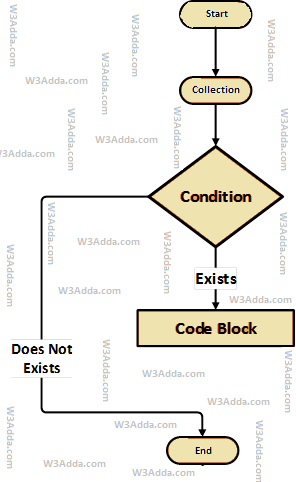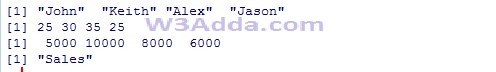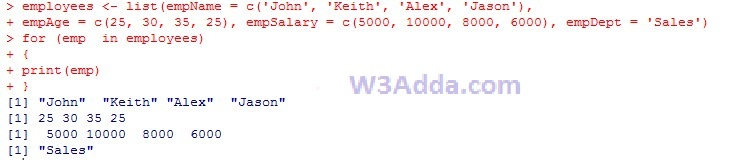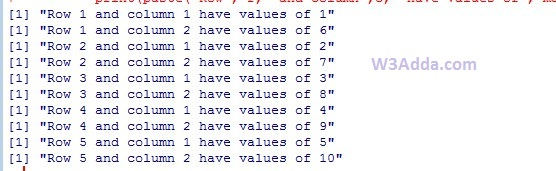# R for loop

In this tutorial you will learn about the R for loop and its application with practical example.

## R for loop

The for in loop takes a list or collection of data(list, data frame, vector, matrix , or anything that provides an iterator) and iterate through the its elements one at a time in sequence.

## Swift For In Loop Flow DiagramSyntax:-

Here, items is a vector that allows us to fetch each of the single element, and item hold the the current element fetched from the items.

## Iterating over a Vector using for loop

In R, we can loop over a vector using for loop as following –

Example:-

Here, we have initialized a vector employees with 4 elements and then loop over it using for loop. When we run the above R script, we see the following output –

Output:-## Iterating over a list using for loop

In R, we can loop over a list using for loop as following –

Example:-

Here, we have created a list employees with four vectors empName, empAge, empSalary and empDept. Next, we are iterating over it.

When we run the above R script, we see the following output –

Output:-## Iterating over a matrix using for loop

In R, we can loop over a matrix using for loop as following –

Example:-

Here, we have [5,2] matrix with 5 row 2 columns. In order to iterating over its elements we would need two for loops, one for rows and another one for columns.

Output:-In this tutorial we have learn about the R for loop and its application with practical example. I hope you will like this tutorial.